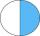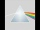# Equations with Fractions problems

#### Number of problems found: 211

• Equation with fractionsSolve an equation: ? It is an equation with fractions.
• Simple equation 5Solve equation with fractions: X × 3/8 = 1/2
• EqnSolve equation with fractions: 2x/3-50=40+x/4
• Equation with mixed fractions2 3/5 of 1430+? = 1900. How to do this problem
• Eq-fracSolve the following equation with fractions: h + 1/3 =5/3
• Equation 15Solve equation with variables on both sides:
• Equation 20In given equation: 8/9-4/5=2/9+x, find x
• Simple equation 6Solve equation with one variable: X/2+X/3+X/4=X+4
• Equation with xSolve the following equation: 2x- (8x + 1) - (x + 2) / 5 = 9
• Simple equation 2Find X in this simple equation: X/9 = 96/108
• FractionsThree-quarters of an unknown number are 4/5. What is 5/6 of this unknown number?
• EquationFind x: x + 1/2 = 1/3
• Simple equation 324 = n • 27, solve for n
• Equation - inverseSolve for x: 7: x = 14: 1000
• Fraction + eqSolve following simple equation with fractions: -5/6(8+5b) = 75 + 5/3b
• Reciprocal equation 3Solve reciprocal equation: 1/2 + 2/3=1/x
• EquationSolve equation and check the result: 1.4x - 3/2 + x - 9,8 = x + 0,4/3 - 7 + 1,6/6
• DenominatorCalculate the missing denominator x: ?
• Unknown numberI think the number - its sixth is 3 smaller than its third.Please determine the solvability conditions of the equation, solve the equation and perform the test: x divided by x squared minus 2x plus1 the whole minus x + 3 divided by x squared minus 1 this is equal to 0: x/(x2-2x+1) - (x+3)/( x2-1) = 0